# NMath User's Guide

17.4 Hermitian Matrices (.NET, C#, CSharp, VB, Visual Basic, F#)

A Hermitian matrix is a square matrix which satisfieswhere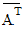denotes the conjugate transpose of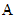. That is,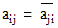for all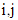, where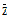denotes the complex conjugate. (The conjugate of a complex number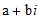is defined as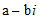.) For example, this is a 4 x 4 Hermitian matrix: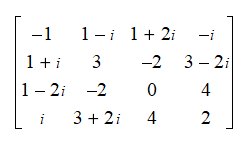According to the strict definition of a Hermitian matrix, the diagonal elements must be real numbers, since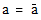only for real numbers, while other elements may be complex. NMath relaxes this requirement and permits complex elements on the diagonal. The provided MakeDiagonalReal() method sets the imaginary parts on the main diagonal to zero, thereby meeting the strict definition of a Hermitian matrix.

NMath provides Hermitian matrix classes for single- and double-precision complex numbers. The classnames are and . A symmetric matrix is a special case of a Hermitian matrix where all the elements are real (Section 17.3).

For efficiency, only the upper triangle is stored. The storage scheme is the same as for an upper triangular matrix (Section 17.2).

Top

Top